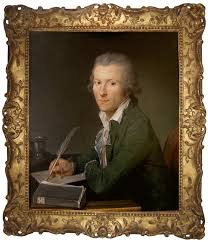# Figure

Figure A is a scale image of Figure B, as shown. (figure a is 6 inches and figure b is x inches, both squares) The scale that maps Figure A onto Figure B is 1:1 1/3. Enter the value f x

x =  8 in

### Step-by-step explanation:Did you find an error or inaccuracy? Feel free to write us. Thank you!

Tips for related online calculators
Need help with mixed numbers? Try our mixed-number calculator.
Check out our ratio calculator.
Do you want to convert length units?

#### Grade of the word problem:

We encourage you to watch this tutorial video on this math problem: